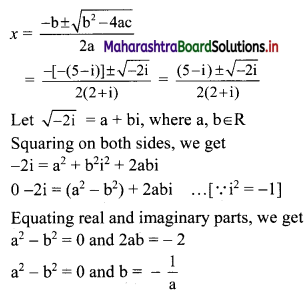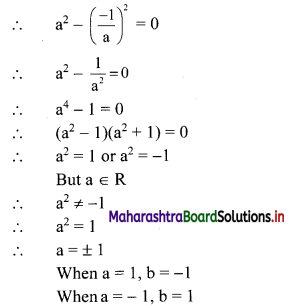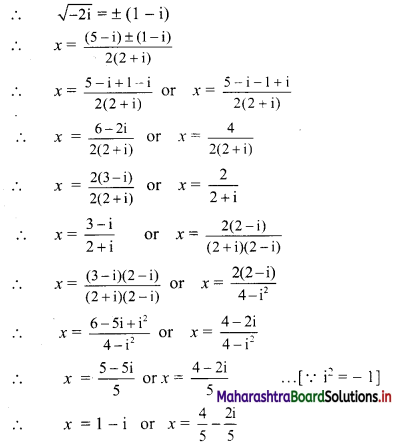# Maharashtra Board 11th Commerce Maths Solutions Chapter 3 Complex Numbers Ex 3.2

Balbharati Maharashtra State Board 11th Commerce Maths Solution Book Pdf Chapter 3 Complex Numbers Ex 3.2 Questions and Answers.

## Maharashtra State Board 11th Commerce Maths Solutions Chapter 3 Complex Numbers Ex 3.2

Question 1.
Find the square root of the following complex numbers:
(i) -8 – 6i
Solution:
Let $$\sqrt{-8-6 i}$$ = a + bi, where a, b ∈ R
Squaring on both sides, we get
-8 – 6i = (a + bi)2
-8 – 6i = a2 + b2i2 + 2abi
-8 – 6i = (a2 – b2) + 2abi …..[∵ i2 = -1]
Equating real and imaginary parts, we get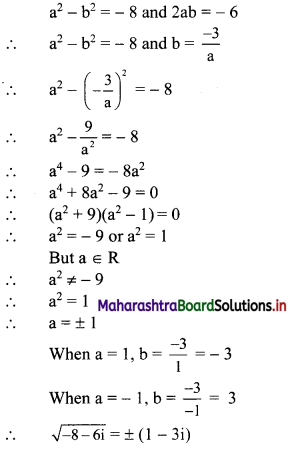(ii) 7 + 24i
Solution:
Let $$\sqrt{7+24 i}$$ = a + bi, where a, b ∈ R
Squaring on both sides, we get
7 + 24i = (a + bi)2
7 + 24i = a2 + b2i2 + 2abi
7 + 24i = (a2 – b2) + 2abi …..[∵ i2 = -1]
Equating real and imaginary parts, we get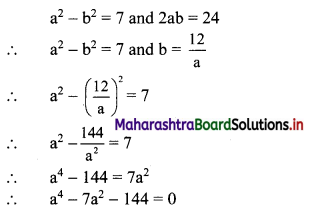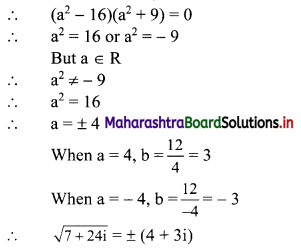(iii) 1 + 4√3i
Solution:
Let $$\sqrt{1+4 \sqrt{3} i}$$ = a + bi, where a, b ∈ R
Squaring on both sides, we get
1 + 4√3i = (a + bi)2
1 + 4√3i = a2 + b2i2 + 2abi
1 +4√3i = (a2 – b2) + 2abi ……[∵ i2 = -1]
Equating real and imaginary parts, we get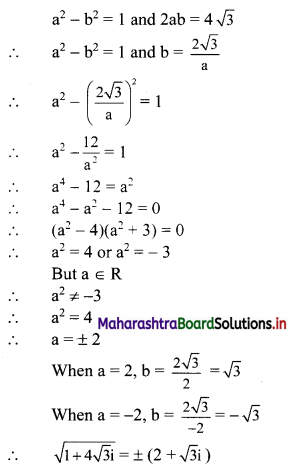(iv) 3 + 2√10i
Solution:
Let $$\sqrt{3+2 \sqrt{10}} i$$ = a + bi, where a, b ∈ R
Squaring on both sides, we get
3 + 2√10i = (a + bi)2
3 + 2√10i = a2 + b2i2 + 2abi
3 + 2√10i = (a2 – b2) + 2abi …..[∵ i2 = -1]
Equating real and imaginary parts, we get
a2 – b2 = 3 and 2ab = 2√10
a2 – b2 = 3 and b = $$\frac{\sqrt{10}}{a}$$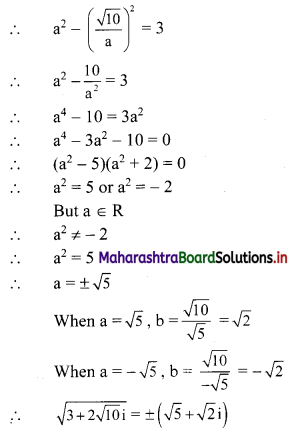(v) 2(1 – √3i)
Solution:
Let $$\sqrt{2(1-\sqrt{3} i)}$$ = a + bi, where a, b ∈ R
Squaring on both sides, we get
2(1 – √3i) = (a + bi)2
2(1 – √3i) = a2 + b2i2 + 2abi
2 – 2√3i = (a2 – b2) + 2abi …..[∵ i2 = -1]
Equating real and imaginary parts, we get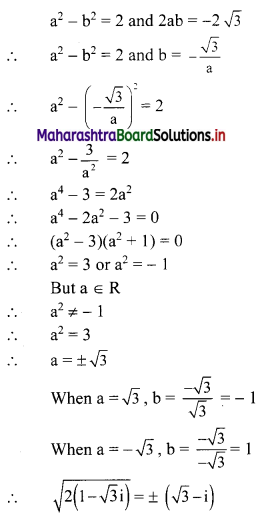Question 2.
(i) 8x2 + 2x + 1 = 0
Solution:
Given equation is 8x2 + 2x + 1 = 0
Comparing with ax2 + bx + c = 0, we get
a = 8, b = 2, c = 1
Discriminant = b2 – 4ac
= (2)2 – 4 × 8 × 1
= 4 – 32
= -28 < 0
So, the given equation has complex roots.
These roots are given by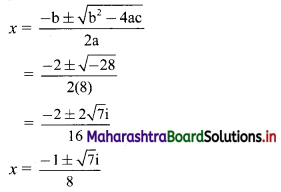∴ the roots of the given equation are $$\frac{-1+\sqrt{7} \mathrm{i}}{8}$$ and $$\frac{-1-\sqrt{7} \mathrm{i}}{8}$$

(ii) 2x2 – √3x + 1 = 0
Solution:
Given equation is 2x2 – √3x + 1 = 0
Comparing with ax2 + bx + c = 0, we get
a = 2, b = -√3, c = 1
Discriminant = b2 – 4ac
= (-√3)2 – 4 × 2 × 1
= 3 – 8
= -5 < 0
So, the given equation has complex roots.
These roots are given by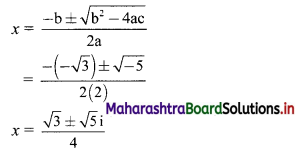∴ the roots of the given equation are $$\frac{\sqrt{3}+\sqrt{5} i}{4}$$ and $$\frac{\sqrt{3}-\sqrt{5} i}{4}$$

(iii) 3x2 – 7x + 5 = 0
Solution:
Given equation is 3x2 – 7x + 5 = 0
Comparing with ax2 + bx + c = 0, we get
a = 3, b = -7, c = 5
Discriminant = b2 – 4ac
= (-7)2 – 4 × 3 × 5
= 49 – 60
= -11 < 0
So, the given equation has complex roots.
These roots are given by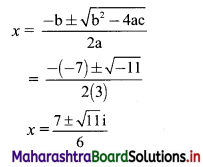∴ the roots of the given equation are $$\frac{7+\sqrt{11} i}{6}$$ and $$\frac{7-\sqrt{11} i}{6}$$

(iv) x2 – 4x + 13 = 0
Solution:
Given equation is x2 – 4x + 13 = 0
Comparing with ax2 + bx + c = 0, we get
a = 1, b = -4, c = 13
Discriminant = b2 – 4ac
= (-4)2 – 4 × 1 × 13
= 16 – 52
= -36 < 0
So, the given equation has complex roots.
These roots are given by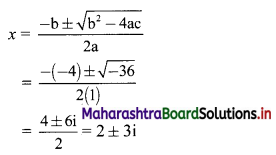∴ the roots of the given equation are 2 + 3i and 2 – 3i.Question 3.
(i) x2 + 3ix + 10 = 0
Solution:
Given equation is x2 + 3ix + 10 = 0
Comparing with ax2 + bx + c = 0, we get
a = 1, b = 3i, c = 10
Discriminant = b2 – 4ac
= (3i)2 – 4 × 1 × 10
= 9i2 – 40
= -9 – 40 …..[∵ i2 = -1]
= -49
So, the given equation has complex roots.
These roots are given by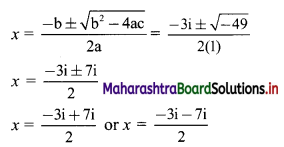∴ x = 2i or x = -5i
∴ the roots of the given equation are 2i and -5i.
Check:
If x = 2i and x = -5i satisfy the given equation, then our answer is correct.
L.H.S. = x2 + 3ix + 10
= (2i)2 + 3i(2i) + 10i
= 4i2 + 6i2 + 10
= 10i2 + 10
= -10 + 10 ……[∵ i2 = -1]
= 0
= R.H.S.
L.H.S. = x2 + 3ix + 10
= (-5i)2 + 3i(-5i) + 10
= 25i2 – 15i2 + 10
= 10i2 + 10
= -10 + 10 …..[∵ i2 = -1]
= 0
= R.H.S.

(ii) 2x2 + 3ix + 2 = 0
Solution:
Given equation is 2x2 + 3ix + 2 = 0
Comparing with ax2 + bx + c = 0, we get
a = 2, b = 3i, c = 2
Discriminant = b2 – 4ac
= (3i)2 – 4 × 2 × 2
= 9i2 – 16
= -9 – 16
= -25 < 0
So, the given equation has complex roots.
These roots are given by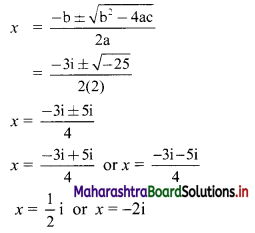∴ the roots of the given equation are $$\frac{1}{2}$$i and -2i.

(iii) x2 + 4ix – 4 = 0
Solution:
Given equation is x2 + 4ix – 4 = 0
Comparing with ax2 + bx + c = 0, we get
a = 1, b = 4i, c = -4
Discriminant = b2 – 4ac
= (4i)2 – 4 × 1 × -4
= 16i2 + 16
= -16 + 16 …..[∵ i2 = -1]
= 0
So, the given equation has equal roots.
These roots are given by∴ the roots of the given equation are -2i and -2i.

(iv) ix2 – 4x – 4i = 0
Solution:
ix2 – 4x – 4i = 0
Multiplying throughout by i, we get
i2x2 – 4ix – 4i2 = 0
∴ -x2 – 4ix + 4 = 0 ……[∵ i2 = -1]
∴ x2 + 4ix – 4 = 0
Comparing with ax2 + bx + c = 0, we get
a = 1, b = 4i, c = -4
Discriminant = b2 – 4ac
= (4i)2 – 4 × 1 × -4
= 16i2 + 16
= -16 + 16 …..[∵ i2 = -1]
= 0
So, the given equation has equal roots.
These roots are given by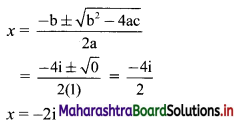∴ the roots of the given equation are -2i and -2i.Question 4.
(i) x2 – (2 + i) x – (1 – 7i) = 0
Solution:
Given equation is x2 – (2 + i)x – (1 – 7i) = 0
Comparing with ax2 + bx + c = 0, we get
a = 1, b = -(2 + i), c = -(1 – 7i)
Discriminant = b2 – 4ac
= [-(2 + i)]2 – 4 × 1 × -(1 – 7i)
= 4 + 4i + i2 + 4 – 28i
= 4 + 4i – 1 + 4 – 28i …….[∵ i2 = -1]
= 7 – 24i
So, the given equation has complex roots.
These roots are given by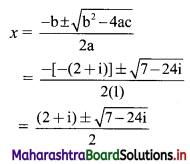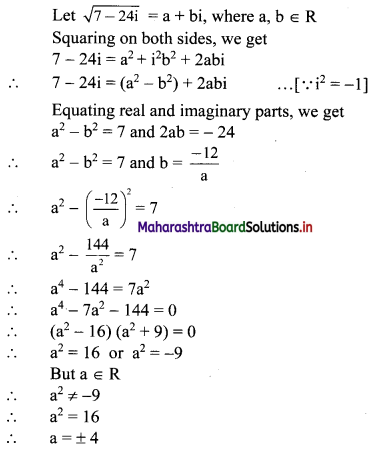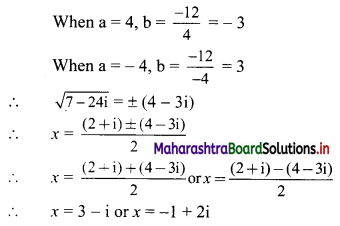(ii) x2 – (3√2 + 2i) x + 6√2i = 0
Solution:
Given equation is x2 – (3√2 + 2i) x + 6√2i = 0
Comparing with ax2 + bx + c = 0, we get
a = 1, b = -(3√2 + 2i), c = 6√2i
Discriminant = b2 – 4ac
= [-(3√2 + 2i)]2 – 4 × 1 × 6√2i
= 18 + 12√2i + 4i2 – 24√2i
= 18 – 12√2i – 4 …..[∵ i2 = -1]
= 14 – 12√2i
So, the given equation has complex roots.
These roots are given by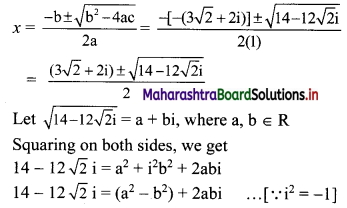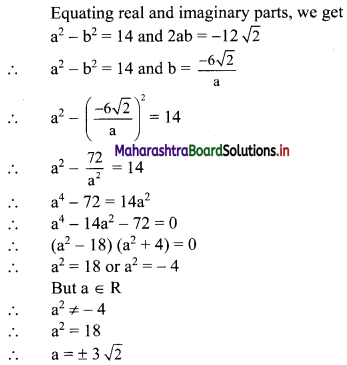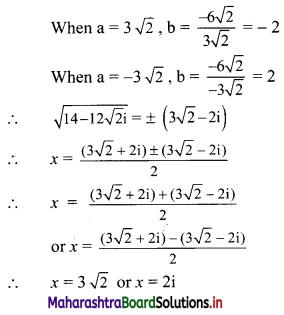(iii) x2 – (5 – i) x + (18 + i) = 0
Solution:
Given equation is x2 – (5 – i)x + (18 + i) = 0
Comparing with ax2 + bx + c = 0, we get
a = 1, b = -(5 – i), c = 18 + i
Discriminant = b2 – 4ac
= [-(5 – i)]2 – 4 × 1 × (18 + i)
= 25 – 10i + i2 – 72 – 4i
= 25 – 10i – 1 – 72 – 4i …..[∵ i2 = -1]
= -48 – 14i
So, the given equation has complex roots.
These roots are given by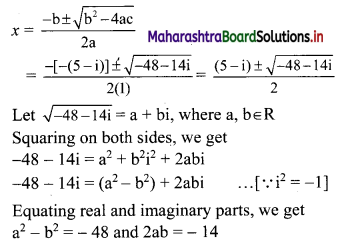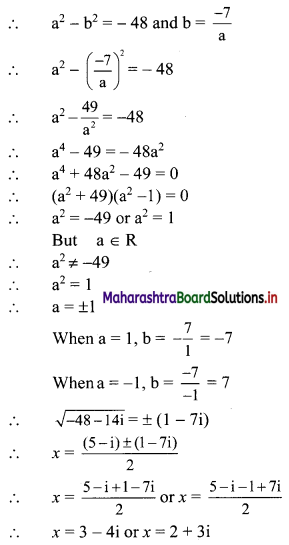(iv) (2 + i) x2 – (5 – i) x + 2(1 – i) = 0
Solution:
Given equation is
(2 + i) x2 – (5 – i) x + 2(1 – i) = 0
Comparing with ax2 + bx + c = 0, we get
a = 2 + i, b = -(5 – i), c = 2(1 – i)
Discriminant = b2 – 4ac
= [-(5 – i)]2 – 4 × (2 + i) × 2(1 – i)
= 25 – 10i + i2 – 8(2 + i)(1 – i)
= 25 – 10i + i2 – 8(2 – 2i + i – i2)
= 25 – 10i – 1 – 8(2 – i + 1) …..[∵ i2 = -1]
= 25 – 10i – 1 – 16 + 8i – 8
= -2i
So, the given equation has complex roots.
These roots are given by